Ex 6.3

Chapter 6 Class 12 Application of Derivatives
Serial order wise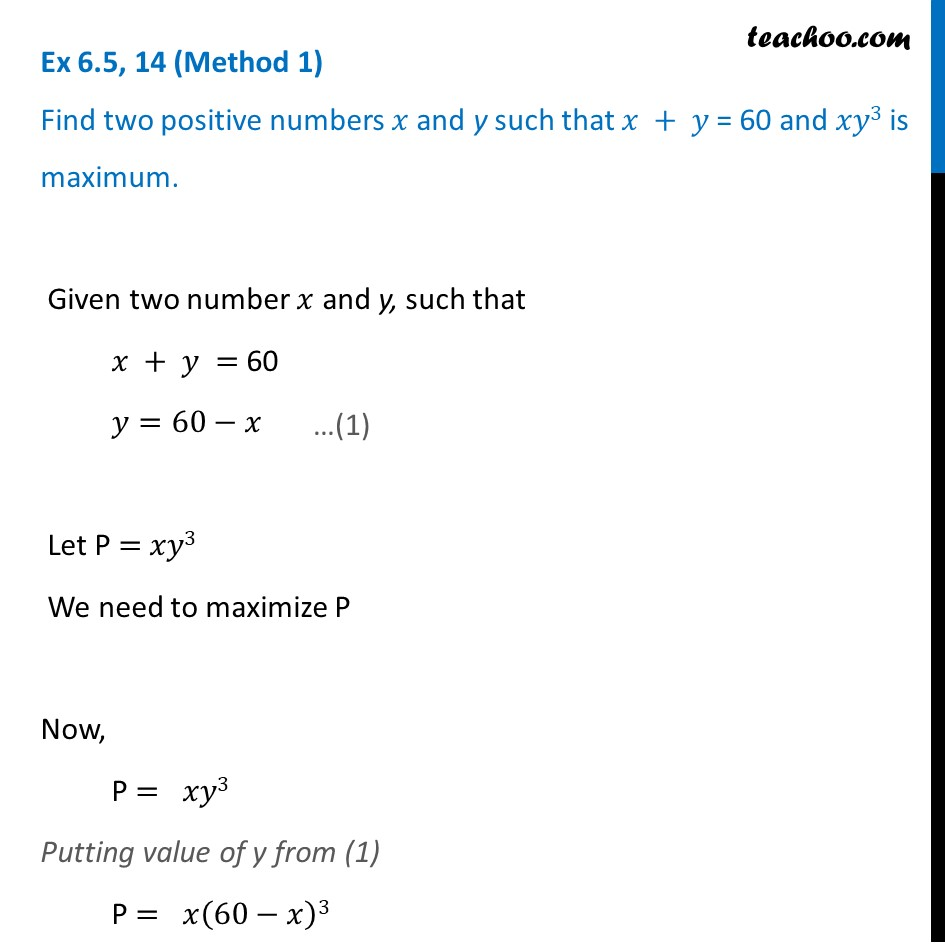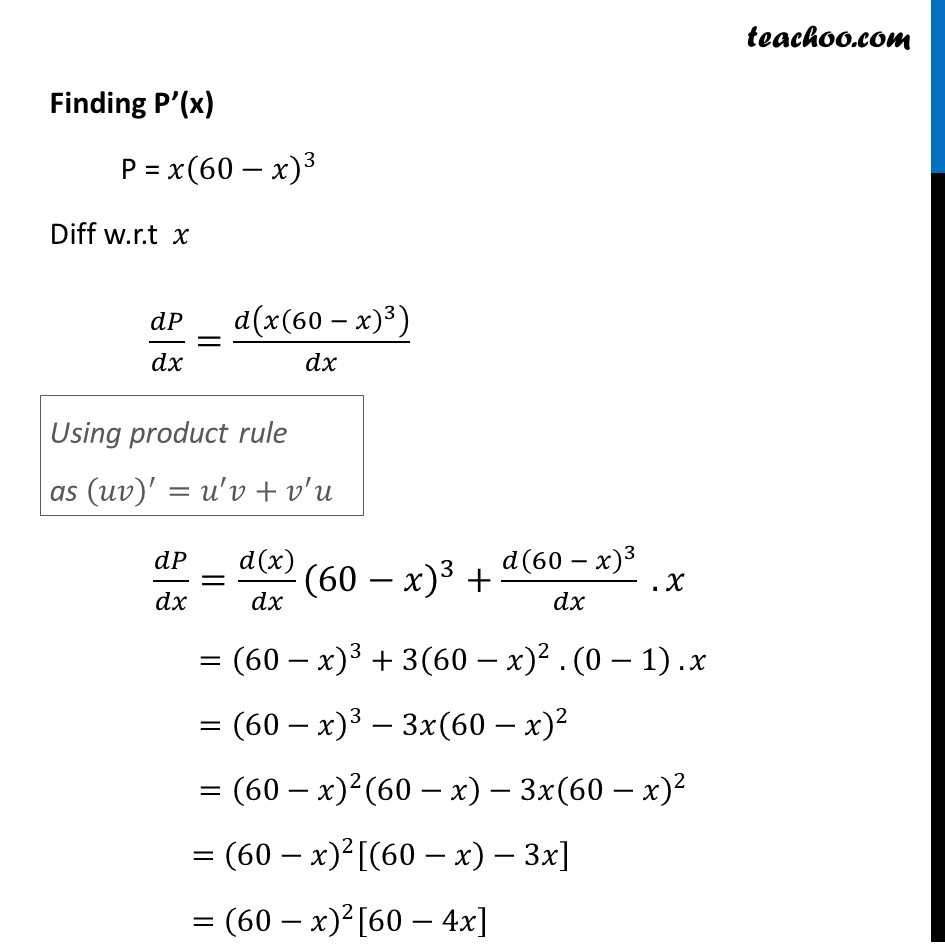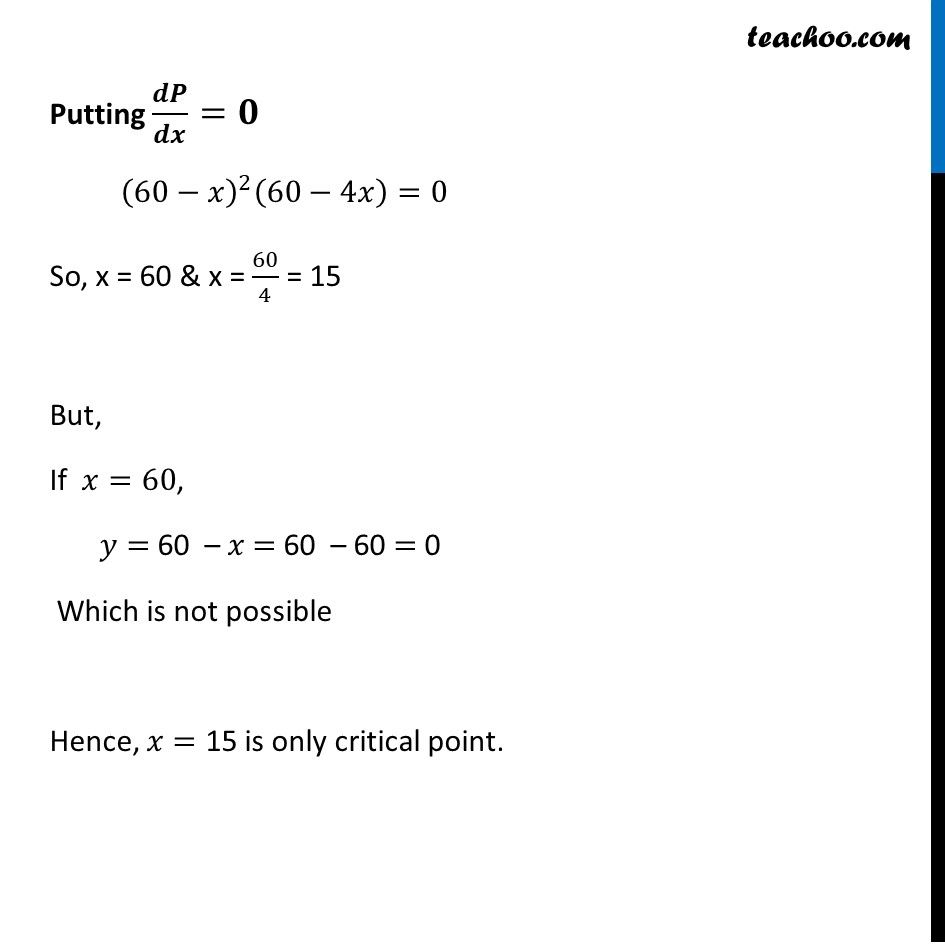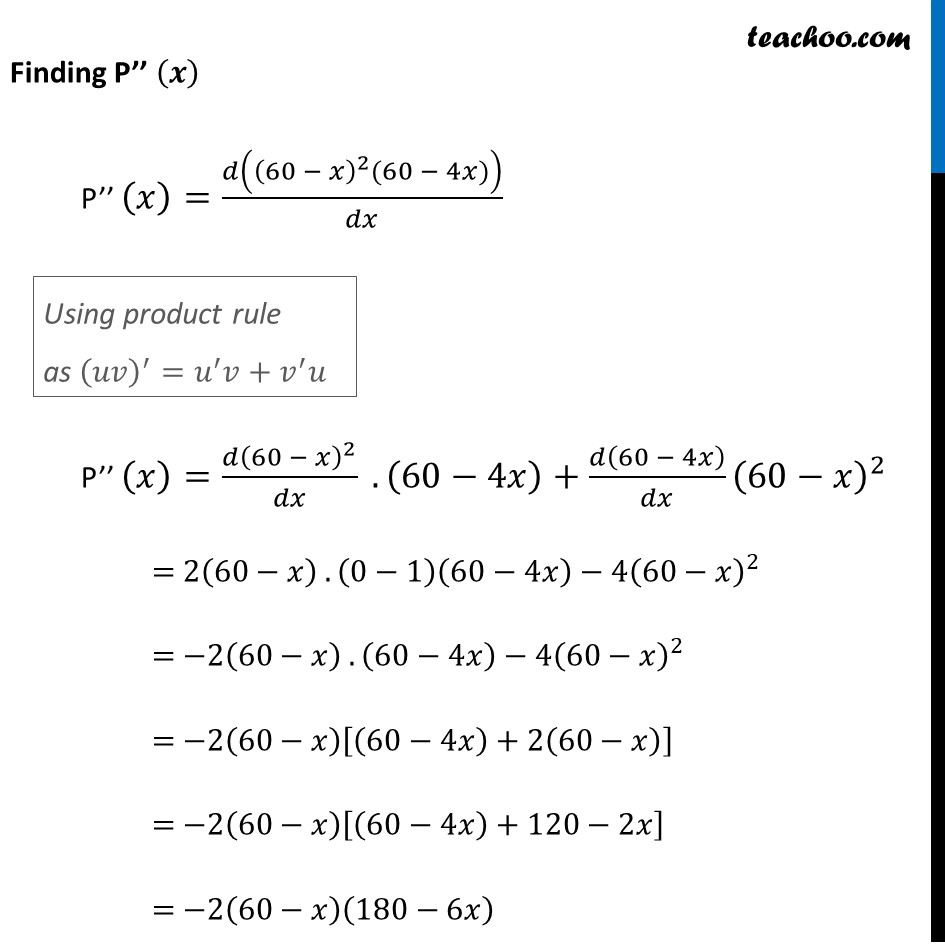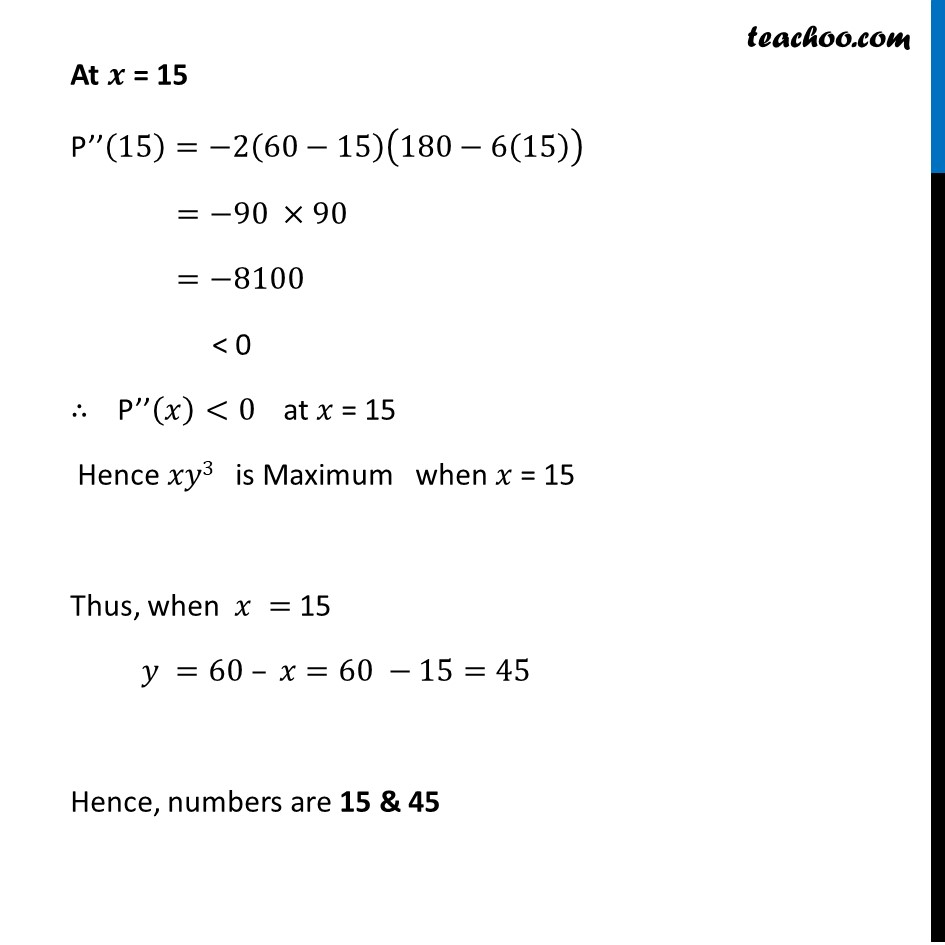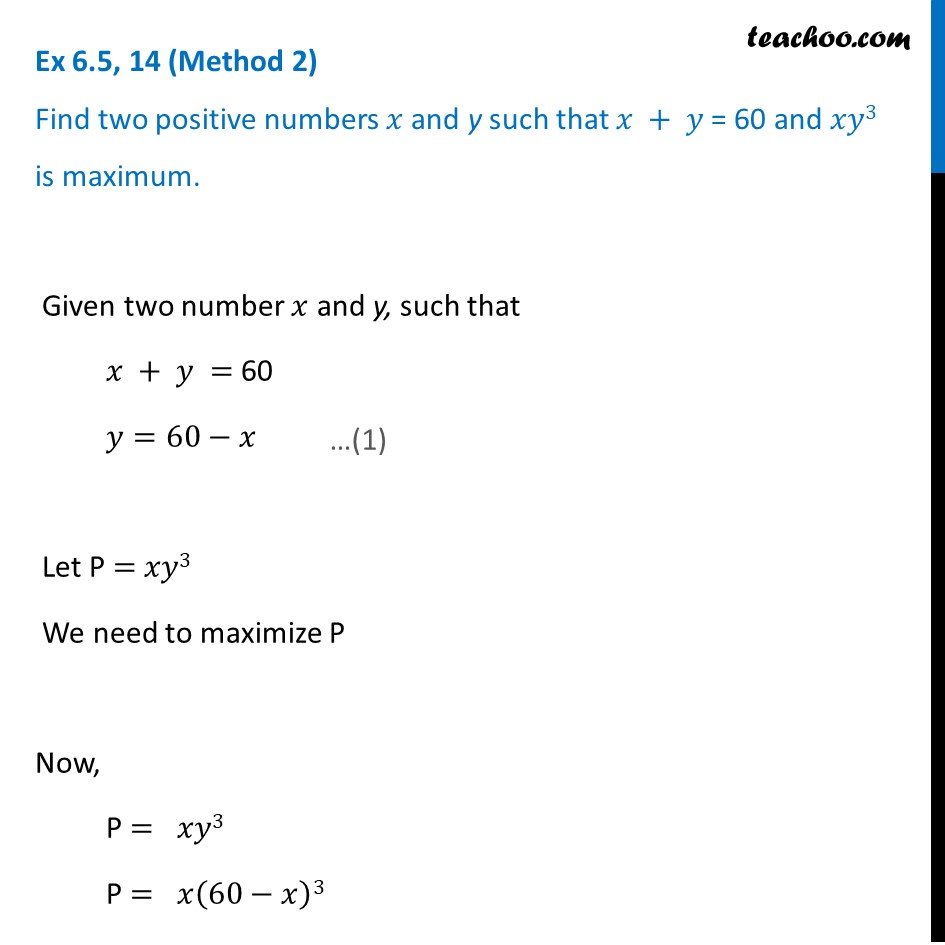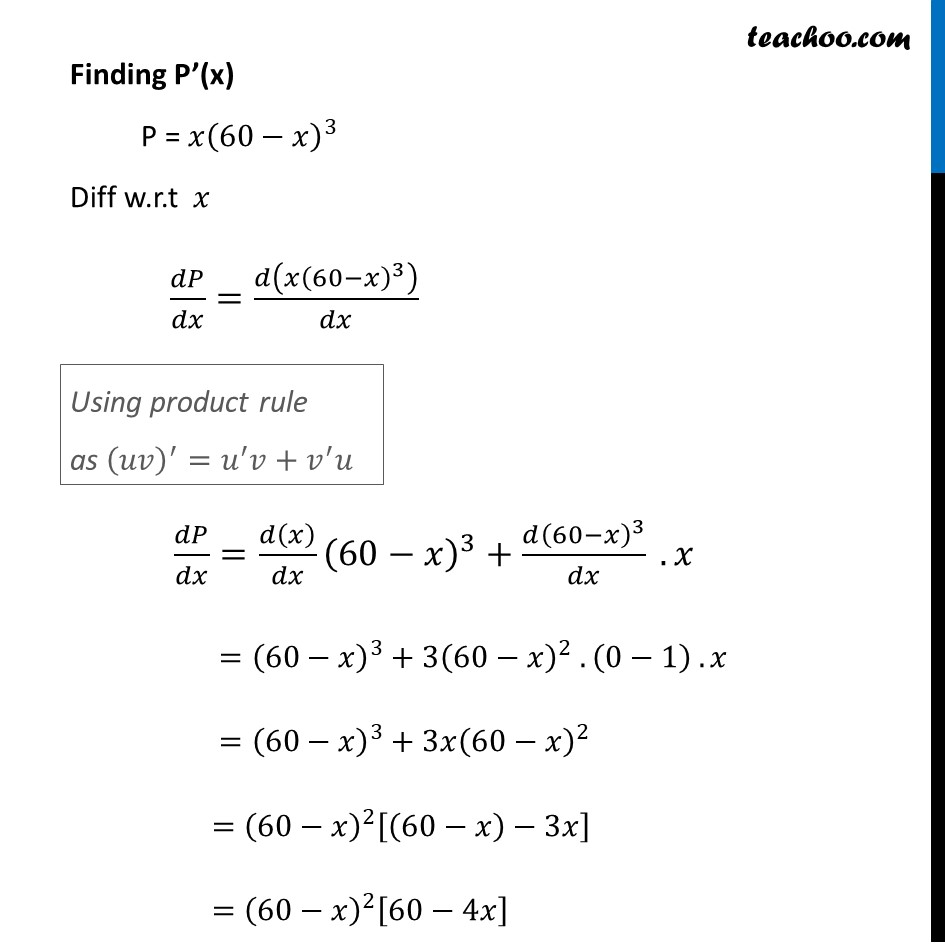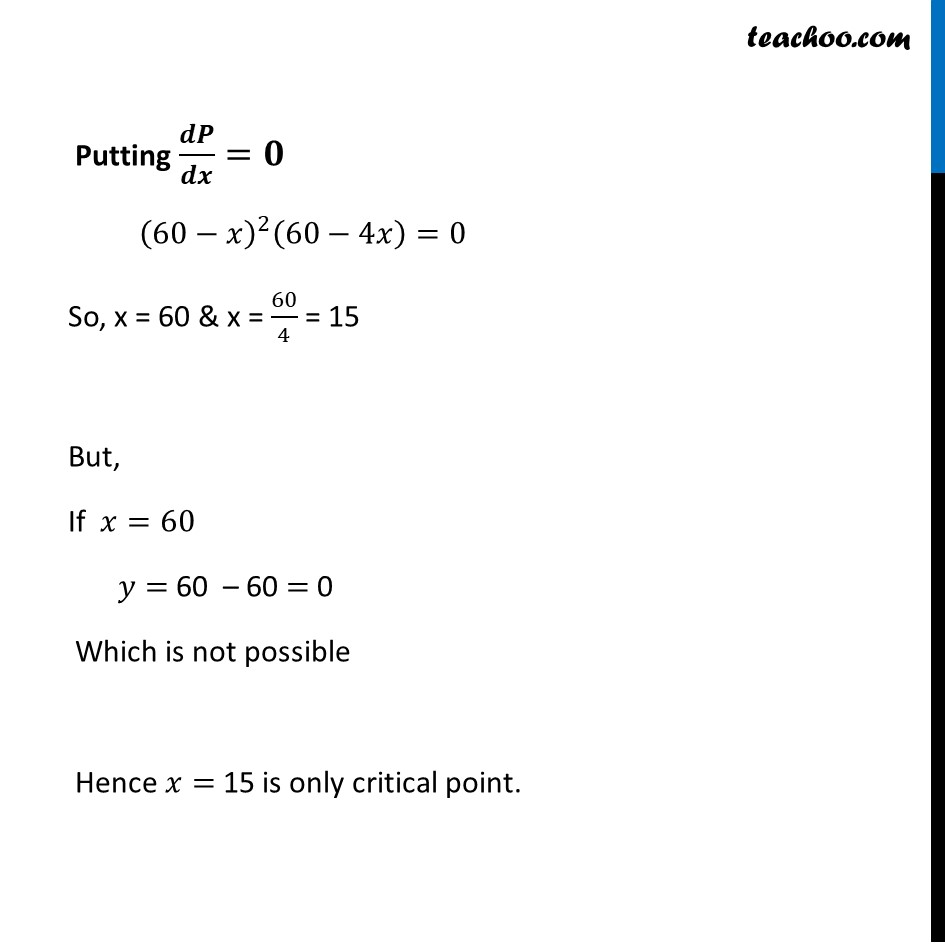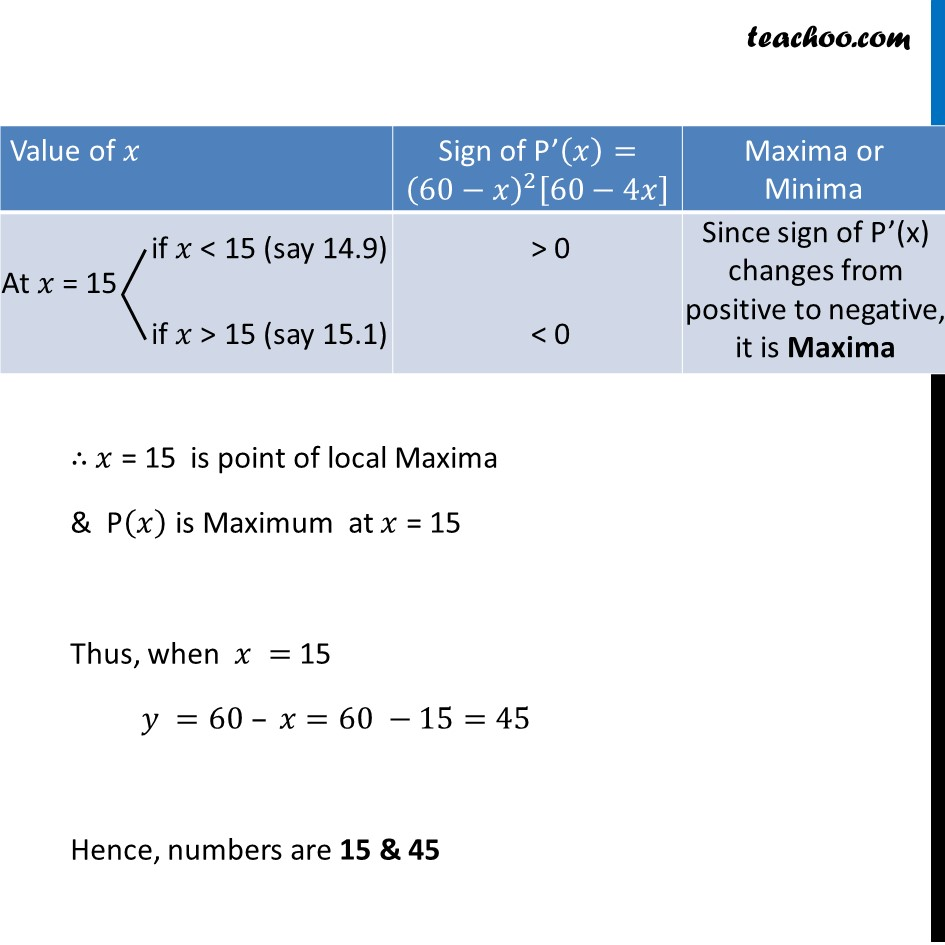Learn in your speed, with individual attention - Teachoo Maths 1-on-1 Class

### Transcript

Ex 6.3, 14 (Method 1) Find two positive numbers 𝑥 and y such that 𝑥 + 𝑦 = 60 and 𝑥𝑦3 is maximum. Given two number 𝑥 and y, such that 𝑥 + 𝑦 = 60 𝑦=60−𝑥 Let P = 𝑥𝑦3 We need to maximize P Now, P = 𝑥𝑦3 Putting value of y from (1) P = 𝑥(60−𝑥)3 Finding P’(x) P = 𝑥(60−𝑥)^3 Diff w.r.t 𝑥 𝑑𝑃/𝑑𝑥=𝑑(𝑥(60 − 𝑥)^3 )/𝑑𝑥 𝑑𝑃/𝑑𝑥=𝑑(𝑥)/𝑑𝑥 (60−𝑥)^3+(𝑑(60 − 𝑥)^3)/𝑑𝑥 . 𝑥 =(60−𝑥)^3+〖3(60−𝑥)〗^2 . (0−1) . 𝑥 =(60−𝑥)^3−3𝑥(60−𝑥)^2 =(60−𝑥)^2 (60−𝑥)−3𝑥(60−𝑥)^2 =(60−𝑥)^2 [(60−𝑥)−3𝑥] =(60−𝑥)^2 [60−4𝑥] Finding P’(x) P = 𝑥(60−𝑥)^3 Diff w.r.t 𝑥 𝑑𝑃/𝑑𝑥=𝑑(𝑥(60 − 𝑥)^3 )/𝑑𝑥 𝑑𝑃/𝑑𝑥=𝑑(𝑥)/𝑑𝑥 (60−𝑥)^3+(𝑑(60 − 𝑥)^3)/𝑑𝑥 . 𝑥 =(60−𝑥)^3+〖3(60−𝑥)〗^2 . (0−1) . 𝑥 =(60−𝑥)^3−3𝑥(60−𝑥)^2 =(60−𝑥)^2 (60−𝑥)−3𝑥(60−𝑥)^2 =(60−𝑥)^2 [(60−𝑥)−3𝑥] =(60−𝑥)^2 [60−4𝑥] Using product rule as (𝑢𝑣)^′=𝑢^′ 𝑣+𝑣^′ 𝑢 Putting 𝒅𝑷/𝒅𝒙=𝟎 (60−𝑥)^2 (60−4𝑥)=0 So, x = 60 & x = 60/4 = 15 But, If 𝑥=60, 𝑦= 60 – 𝑥 = 60 – 60 = 0 Which is not possible Hence, 𝑥= 15 is only critical point. Finding P’’ (𝒙) P’’ (𝑥)=𝑑((60 − 𝑥)^2 (60 − 4𝑥))/𝑑𝑥 P’’ (𝑥)=(𝑑(60 − 𝑥)^2)/𝑑𝑥 . (60−4𝑥)+𝑑(60 − 4𝑥)/𝑑𝑥 (60−𝑥)^2 = 2(60−𝑥) .(0−1)(60−4𝑥)−4(60−𝑥)^2 = −2(60−𝑥) . (60−4𝑥)−4(60−𝑥)^2 = −2(60−𝑥)[(60−4𝑥)+2(60−𝑥)] = −2(60−𝑥)[(60−4𝑥)+120−2𝑥] = −2(60−𝑥)(180−6𝑥) Using product rule as (𝑢𝑣)^′=𝑢^′ 𝑣+𝑣^′ 𝑢 At 𝒙 = 15 P’’(15)=−2(60−15)(180−6(15)) =−90 ×90 =−8100 < 0 ∴ P’’(𝑥)<0 at 𝑥 = 15 Hence 𝑥𝑦3 is Maximum when 𝑥 = 15 Thus, when 𝑥 = 15 𝑦 =60 – 𝑥=60 −15=45 Hence, numbers are 15 & 45 Ex 6.3, 14 (Method 2) Find two positive numbers 𝑥 and y such that 𝑥 + 𝑦 = 60 and 𝑥𝑦3 is maximum. Given two number 𝑥 and y, such that 𝑥 + 𝑦 = 60 𝑦=60−𝑥 Let P = 𝑥𝑦3 We need to maximize P Now, P = 𝑥𝑦3 P = 𝑥(60−𝑥)3 Finding P’(x) P = 𝑥(60−𝑥)^3 Diff w.r.t 𝑥 𝑑𝑃/𝑑𝑥=𝑑(𝑥(60−𝑥)^3 )/𝑑𝑥 𝑑𝑃/𝑑𝑥=𝑑(𝑥)/𝑑𝑥 (60−𝑥)^3+(𝑑(60−𝑥)^3)/𝑑𝑥 . 𝑥 =(60−𝑥)^3+〖3(60−𝑥)〗^2 . (0−1) . 𝑥 =(60−𝑥)^3+3𝑥(60−𝑥)^2 =(60−𝑥)^2 [(60−𝑥)−3𝑥] =(60−𝑥)^2 [60−4𝑥] Using product rule as (𝑢𝑣)^′=𝑢^′ 𝑣+𝑣^′ 𝑢 Putting 𝒅𝑷/𝒅𝒙=𝟎 (60−𝑥)^2 (60−4𝑥)=0 So, x = 60 & x = 60/4 = 15 But, If 𝑥=60 𝑦= 60 – 60 = 0 Which is not possible Hence 𝑥= 15 is only critical point. ∴ 𝑥 = 15 is point of local Maxima & P(𝑥) is Maximum at 𝑥 = 15 Thus, when 𝑥 = 15 𝑦 =60 – 𝑥=60 −15=45 Hence, numbers are 15 & 45